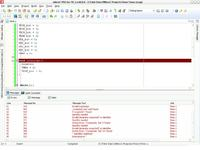# [SOLVED]PIC16F887 Interrupt Problem

Status
Not open for further replies.

#### tahir4awan

##### Full Member level 4
I wrote this code just to check how interrupt works but the problem is MikroC is not compiling the code

----------------------------------------------------------------------------
int count = 0;
void main()
{
ANSEL = 0;
ANSELH = 0;
TRISB = 0x00;
GIE_bit = 1;
PEIE_bit = 1;
T0IE_bit = 1;
T0IF_bit = 0;
T0CS_bit = 1;
T0SE_bit = 0;
PSA_bit = 0;
PS0_bit = 1;
PS1_bit = 1;
PS2_bit = 1;
TMR0 = 0;

void interrupt() {
count++;
TMR0 = 0;
T0IF_bit = 0;
}

while(1){
if(count==5)
{
PORTB = 0x01;
}
else if(count==10)
{
PORTB = 0x02;
}
else if(count==15)
{
PORTB = 0x03;
}
else if(count==20)
{
PORTB = 0x04;
}
}
}

---------------------------------------------------------------------------can someone tell me what is the problem?

I didn´t see the following command at Interrupt() routine :

Code:
INTCON.INTF = 0;

Neither that at begin :

Code:
    INTCON.GIE = 1; //Enable Global Interrupt
+++

•tahir4awan

### tahir4awan

Points: 2
use interrupt subrutine as "void static interrupt isr(void)"

•tahir4awan

### tahir4awan

Points: 2
Thanks guys I solved the problem using ISR void Interrupt(). But one thing I didn't understand that in MikroC the ISR is simply write as void Interrupt() but many people write it differently, Why?

because syntaxes are may be different in different compilers

•tahir4awan

### tahir4awan

Points: 2
...I solved the problem using ISR void Interrupt()...

ISR statement is not a standard ANSI-C command.
There exists a wide of other commands with specific sintax at each compiler.
Maybe that ISR declaration implicity instructs compiler to add above mentioned instructions to Interrup routine.

+++

•tahir4awan

Points: 2